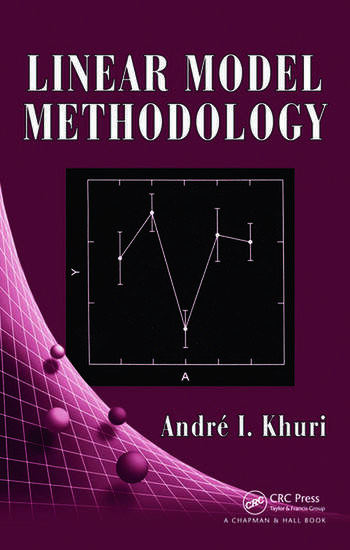# Linear Model Methodology

## 1st Edition

Andre I. Khuri

Chapman and Hall/CRC
Published October 21, 2009
Reference - 562 Pages - 13 B/W Illustrations
ISBN 9781584884811 - CAT# C4819

USD\$150.00

FREE Standard Shipping!

## Preview

### Summary

Given the importance of linear models in statistical theory and experimental research, a good understanding of their fundamental principles and theory is essential. Supported by a large number of examples, Linear Model Methodology provides a strong foundation in the theory of linear models and explores the latest developments in data analysis.

After presenting the historical evolution of certain methods and techniques used in linear models, the book reviews vector spaces and linear transformations and discusses the basic concepts and results of matrix algebra that are relevant to the study of linear models. Although mainly focused on classical linear models, the next several chapters also explore recent techniques for solving well-known problems that pertain to the distribution and independence of quadratic forms, the analysis of estimable linear functions and contrasts, and the general treatment of balanced random and mixed-effects models. The author then covers more contemporary topics in linear models, including the adequacy of Satterthwaite’s approximation, unbalanced fixed- and mixed-effects models, heteroscedastic linear models, response surface models with random effects, and linear multiresponse models. The final chapter introduces generalized linear models, which represent an extension of classical linear models.

Linear models provide the groundwork for analysis of variance, regression analysis, response surface methodology, variance components analysis, and more, making it necessary to understand the theory behind linear modeling. Reflecting advances made in the last thirty years, this book offers a rigorous development of the theory underlying linear models.